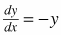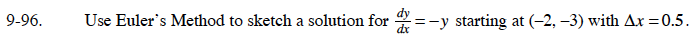### Home > CALC > Chapter 9 > Lesson 9.3.2 > Problem9-96

9-96.

Use Euler's Method to sketch a solution forstarting at (−2, −3) with ∆x = 0.5. Homework Help ✎The initial point is (–2, –3). The next point is (–2 + 0.5, –3 + –(–3)(0.5)) = (–1.5, –1.5).

From (–1.5, –1.5), the next point is (–1.5 + 0.5, –1.5 + –(–1.5)(0.5)) = (–1, –0.75).

Keep repeating this process then sketch the solution using the points.